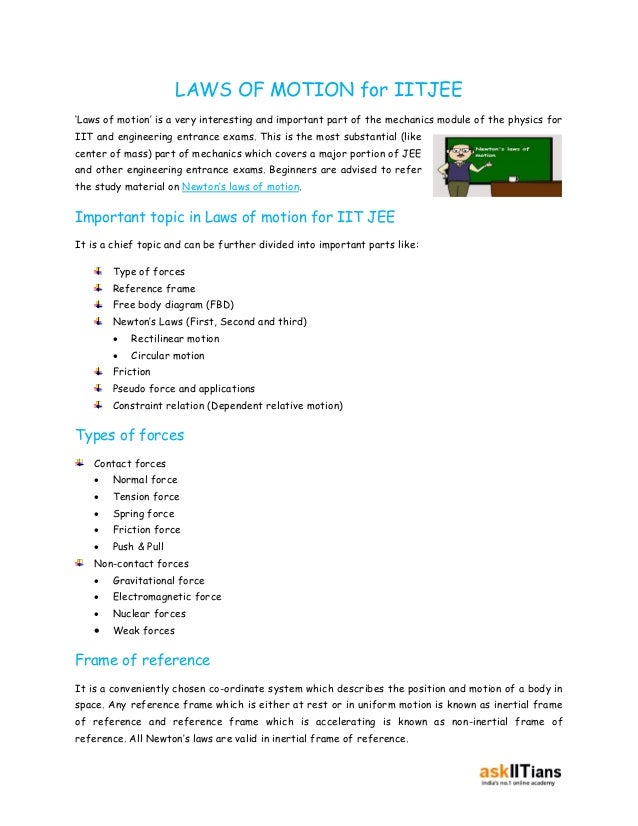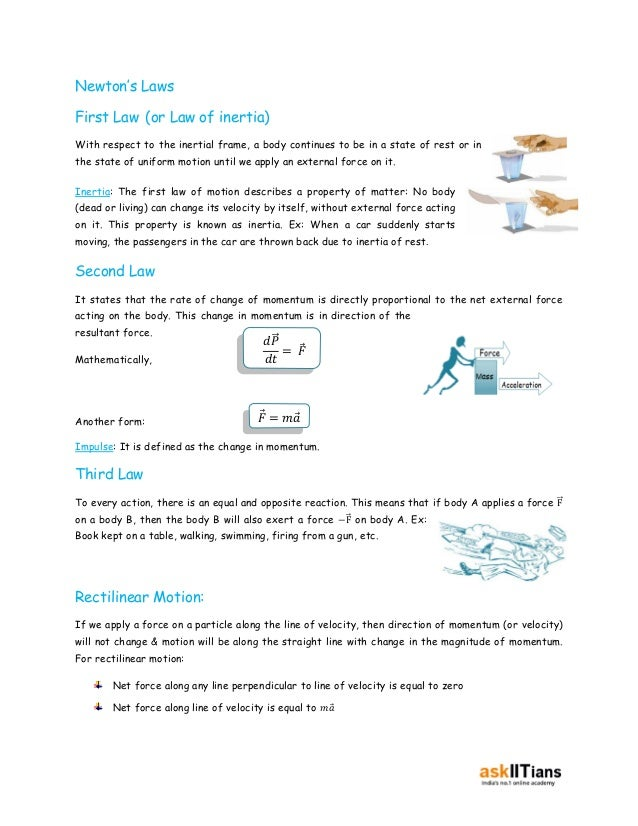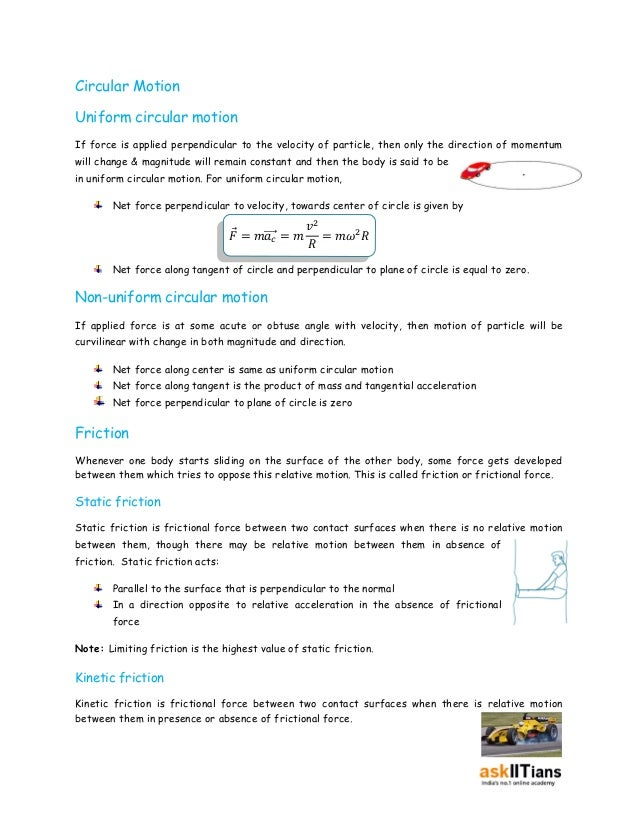Successfully reported this slideshow.You’ve finished this document.
Upcoming SlideShare
AIEEE Fast Problem Solving Tricks
Next

ofUpcoming SlideShare
AIEEE Fast Problem Solving Tricks
Next

Share

# Laws of Motion Preparation Tips for IIT JEE | askIITians

Hone your preparation for IIT JEE Laws of Motion with askIITians best tips. Get top advice from ex-IITians for IIT JEE Laws of Motion preparation.

See all

See all

### Laws of Motion Preparation Tips for IIT JEE | askIITians

1. 1. LAWS OF MOTION for IITJEE ‘Laws of motion’ is a very interesting and important part of the mechanics module of the physics for IIT and engineering entrance exams. This is the most substantial (like center of mass) part of mechanics which covers a major portion of JEE and other engineering entrance exams. Beginners are advised to refer the study material on Newton’s laws of motion. Important topic in Laws of motion for IIT JEE It is a chief topic and can be further divided into important parts like: Type of forces Reference frame Free body diagram (FBD) Newton’s Laws (First, Second and third)  Rectilinear motion  Circular motion Friction Pseudo force and applications Constraint relation (Dependent relative motion) Types of forces Contact forces  Normal force  Tension force  Spring force  Friction force  Push & Pull Non-contact forces  Gravitational force  Electromagnetic force  Nuclear forces  Weak forces Frame of reference It is a conveniently chosen co-ordinate system which describes the position and motion of a body in space. Any reference frame which is either at rest or in uniform motion is known as inertial frame of reference and reference frame which is accelerating is known as non-inertial frame of reference. All Newton’s laws are valid in inertial frame of reference.
2. 2. Newton’s Laws First Law (or Law of inertia) With respect to the inertial frame, a body continues to be in a state of rest or in the state of uniform motion until we apply an external force on it. Inertia: The first law of motion describes a property of matter: No body (dead or living) can change its velocity by itself, without external force acting on it. This property is known as inertia. Ex: When a car suddenly starts moving, the passengers in the car are thrown back due to inertia of rest. Second Law It states that the rate of change of momentum is directly proportional to the net external force acting on the body. This change in momentum is in direction of the resultant force. Mathematically, Another form: 푑푃⃗ 푑푡 = 퐹 퐹 = 푚푎 Impulse: It is defined as the change in momentum. Third Law To every action, there is an equal and opposite reaction. This means that if body A applies a force F⃗ on a body B, then the body B will also exert a force −F⃗ on body A. Ex: Book kept on a table, walking, swimming, firing from a gun, etc. Rectilinear Motion: If we apply a force on a particle along the line of velocity, then direction of momentum (or velocity) will not change & motion will be along the straight line with change in the magnitude of momentum. For rectilinear motion: Net force along any line perpendicular to line of velocity is equal to zero Net force along line of velocity is equal to 푚푎
3. 3. Circular Motion Uniform circular motion If force is applied perpendicular to the velocity of particle, then only the direction of momentum will change & magnitude will remain constant and then the body is said to be in uniform circular motion. For uniform circular motion, Net force perpendicular to velocity, towards center of circle is given by 퐹 = 푚⃗푎⃗⃗⃗푐 = 푚 푣2 푅 = 푚휔2푅 Net force along tangent of circle and perpendicular to plane of circle is equal to zero. Non-uniform circular motion If applied force is at some acute or obtuse angle with velocity, then motion of particle will be curvilinear with change in both magnitude and direction. Net force along center is same as uniform circular motion Net force along tangent is the product of mass and tangential acceleration Net force perpendicular to plane of circle is zero Friction Whenever one body starts sliding on the surface of the other body, some force gets developed between them which tries to oppose this relative motion. This is called friction or frictional force. Static friction Static friction is frictional force between two contact surfaces when there is no relative motion between them, though there may be relative motion between them in absence of friction. Static friction acts: Parallel to the surface that is perpendicular to the normal In a direction opposite to relative acceleration in the absence of frictional force Note: Limiting friction is the highest value of static friction. Kinetic friction Kinetic friction is frictional force between two contact surfaces when there is relative motion between them in presence or absence of frictional force.
4. 4. Magnitude of friction There is no unique or specific relation for static friction force as it is intelligent and self-adjustable force which means that it adjusts its value in such a way that the acceleration of surfaces in contact becomes equal. But there is upper limit of this force beyond which it can’t act and it is the limiting value of static friction which is mathematically given by 퐹푠 ⃗⃗⃗ (푚푎푥) = 휇푠푁 When there is actual relative motion, then kinetic friction is acting and it has a unique value given by Note: Normally, 휇푠 > 휇푘 Angle of Repose 퐹푘 ⃗⃗⃗⃗ = 휇푘푁 The angle of inclination up to which a body can continue to be at rest on the inclined surface without any external force is known as angle of repose. It is dependent on material and nature of surfaces in contact independent of mass of body. Angle of Friction It is the angle between resultant force and normal force to the surface. Constraint Relation Constraint relation is a mathematical equation which relates displacement, velocity and acceleration of two bodies which are in contact or connected by thread, rod and any other thing. Examples: Two bodies connected by string Two rigid bodies in contact Mass-pulley system Pseudo Force Pseudo comes into picture when we describe the motion of a body using non-inertial reference frame. The direction of pseudo force is opposite to the direction of real acceleration of non-inertial reference frame (observer). The magnitude of pseudo force is the product of mass of body and acceleration of non-inertial reference frame. Once we have considered the pseudo force, Newton’s law will give acceleration of particle relative to that reference frame. Centrifugal force is a pseudo force acting on a body from a rotating frame.
5. 5. Is Newton’s Laws of Motion an important part of IIT–JEE preparation? ‘Laws of motion’ is an important part of mechanics which covers 20-25% of JEE physics paper every year. You really need to master the concepts of this chapter to score high in IIT JEE. What are the best books for the preparation of Newton’s laws of motion? Some of the books which are considered to be best for preparation of this section are: Concepts of Physics by H.C. Verma Problem in General Physics by I.E. Irodov Fundamentals of Physics by Halliday, Resnick and Walker Illustration Two particles of mass m each are tied at the ends of a light string of length 2a. The whole system is kept on a frictionless horizontal surface with the string held tight so that each mass is at a distance ‘a’ from the center P (as shown in the figure). Now, the mid-point of the string is pulled vertically upwards with a small but constant force F. As a result, the particles move towards each other on the surface. The magnitude of acceleration, when the separation between them becomes 2x is [IIT-JEE 2007] Solution:
6. 6. Some Interesting Facts When there is static friction between two bodies, then their relative acceleration is zero. Constraint relations are independent of forces and masses of connected bodies. Banking of road is done to ensure safe turning even though friction is negligible. Static fiction value varies from zero to limiting friction value. Real forces are independent of reference frame Graphically, impulse is algebraic area of force time curve We can’t add and subtract forces on two different bodies Newton’s laws are not valid at atomic or microscopic level. Tips to study Laws of motion for IIT JEE Preparation This is the portion of physics where there are chances of committing silly mistakes. You really need to work on your concepts if you want to excel in this chapter. Here are some tips which you must follow to score high in this branch: Do not practice a single problem of laws of motion without drawing a free body diagram. Make a different notebook in which you should write important concepts and formulas on daily basis. Do not read the same concept from different books as it might lead to confusion. Always stick to one particular standard text for your concepts. It is a simple topic but regular practice is essential in order to excel in it. Always follow the basic root to solve the problem rather than jumping to formulas Try your level best to solve each problem before seeing its solution. Practice as many problems as you can from various reference booksas it helps in building up your confidence and enhance your speed. Try to solve previous year JEE questions on laws of motion which would really open your mind and improve your thinking capacity.
7. 7. Practical Applications of Laws of Motion Laws of motion do have infinite number of real life applications. Its applications practically lie in everything that moves. Here are some interesting examples: It’s used extensively in studying the dynamics of rocket, aircrafts, internal combustion engine, orbiting satellites, fire arm, etc. The concepts of the laws of motion are used in many sports like cricket, baseball, football, javelin throw, car racing, etc. Third law has various applications in processes like firing from a gun, fight of jet planes and rockets, walking on sand or ice, rubber ball rebounds from a wall. Concepts of this chapter are used extensively for various road safety purposes like banking of roads. Laws of motion are a substantial part of various industrial applications like machinery, drives, gears, etc.
•#### SarathPerumalla

Sep. 4, 2020
•#### valli6064

Jul. 16, 2019
•#### ShreyaSingh270

Aug. 15, 2018

Total views

6,153

On Slideshare

0

From embeds

0

Number of embeds

4

86

Shares

0